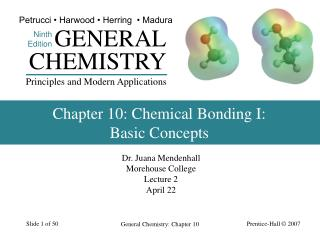# Chapter 10: Chemical Bonding I: Basic Concepts - PowerPoint PPT PresentationDownload PresentationChapter 10: Chemical Bonding I: Basic Concepts

Chapter 10: Chemical Bonding I: Basic ConceptsDownload Presentation## Chapter 10: Chemical Bonding I: Basic Concepts

- - - - - - - - - - - - - - - - - - - - - - - - - - - E N D - - - - - - - - - - - - - - - - - - - - - - - - - - -
##### Presentation Transcript

1. Petrucci • Harwood • Herring • Madura GENERAL Ninth Edition CHEMISTRY Principles and Modern Applications Chapter 10: Chemical Bonding I:Basic Concepts Dr. Juana Mendenhall Morehouse College Lecture 2 April 22 General Chemistry: Chapter 10

2. Objectives • Calculate the formal charge of a ion, compound, or molecule • Draw the electron-pair geometry and molecular shape of a molecule or ions using Valence-shell electron pair repulsion (VSEPR) theory General Chemistry: Chapter 10

3. •• •• O=N=O •• •• 1 FC(O) = 6 - 4 – (4) = 0 2 1 FC(N) = 5 - 0 – (8) = +1 2 Formal Charge A difference b/w the valence e- in an isolated atom & the number of e- assigned to the atom in a Lewis structure 1 FC = #valence e- - #lone pair e- - #bond pair e- 2 + General Chemistry: Chapter 10

4. Formal Charge Rules • For neutral molecules, the sum of the formal charges must add up to zero. (Applies to O3) • For cations, the sum of the formal charges must equal the positive charge. • For anions, the sum of the formal charges must equal the negative charge. General Chemistry: Chapter 10

5. •• •• •• O N O •• 1 FC(O≡) = 6 - 2 – (6) = +1 2 1 FC(O—) = 6 - 6 – (2) = -1 2 1 FC(N) = 5 - 0 – (8) = +1 2 Alternative Lewis Structure + - + •• •• O—N—O •• •• •• •• General Chemistry: Chapter 10

6. Formal charges allow us to write Lewis Structures • For neutral molecules, a Lewis structure in which there are no formal charges is preferable to one in which the formal charges are present. • Lewis structures with the large formal charges (+2,+3, and/or -2,-3, and so on are less plausible than those with small formal charges. • Among Lewis structures having similar distributions of formal charges, the most plausible structure is the one in which negative formal charges are placed on the more electronegative atoms. General Chemistry: Chapter 10

7. - •• + + O≡N—O •• •• •• Alternative Lewis Structures • Sum of FC is the overall charge. • FC should be as small as possible. • Negative FC usually on most electronegative elements. • FC of same sign on adjacent atoms is unlikely. General Chemistry: Chapter 10

8. EXAMPLE Using the Formal Charge in Writing Lewis Structures. Write formal charges for the carbonate ion. General Chemistry: Chapter 10

9. + -½ -½ •• •• •• O O O •• •• Resonance Is one of two or more Lewis structures for a single molecule that cannot be described fully with only one Lewis structure. - + - + •• •• •• •• •• •• O O O O O O •• •• •• •• •• •• General Chemistry: Chapter 10

10. The Shapes of Molecules H O H General Chemistry: Chapter 10

11. Terminology • Bond length – distance between nuclei. • Bond angle – angle between adjacent bonds. • VSEPR Theory • Electron pairs repel each other whether they are in chemical bonds (bond pairs) or unshared (lone pairs). Electron pairs assume orientations about an atom to minimize repulsions. • Electron group geometry – distribution of e- pairs. • Molecular geometry – arrangement of atoms in space. General Chemistry: Chapter 10

12. Balloon Analogy General Chemistry: Chapter 10

13. Methane, Ammonia and Water General Chemistry: Chapter 10

14. Table 10.1 Molecular Geometry as a Function of Electron Group Geometry General Chemistry: Chapter 10

15. General Chemistry: Chapter 10

16. General Chemistry: Chapter 10

17. General Chemistry: Chapter 10

18. General Chemistry: Chapter 10

19. General Chemistry: Chapter 10

20. Applying VSEPR Theory • Draw a plausible Lewis structure. • Determine the number of e- groups and identify them as bond or lone pairs. • Establish the e- group geometry. • Determine the molecular geometry. • Multiple bonds count as one group of electrons. • More than one central atom can be handled individually. General Chemistry: Chapter 10